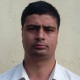Latest Posts
CS/IT MCQ Collections

# Interview Questions on Stack and Queue in Data Structure set-2

This set of multiple choice interview questions on stack and queue in data structure includes an overview of the stack and its implementation. It also includes objective type questions on overview of queues, different types of queues with its operations, and implementation of queues.

1) The queue in which the insertion takes place in the first position after of last element is a ……
A. priority
B. dequeue
C. circular

2) Before inserting into stack one must check the condition ………
A. Overflow
B. Underflow
C. Maximum elements
D. Existing elements

3) The another name of dequeue is ………
A. divided queue
B. distributed queue
C. double ended queue
D. design queue

4) Before deletion condition into stack …… has to be checked.
A. Overflow
B. Underflow
C. Maximum elements
D. Existing elements

5) In dequeue, insertion and deletion takes place of ……….
A. front, rear end
B. only at rear end
C. only at front end
D. both the ends

6) When does Top value of stack change in insertion process?
A. Before insertion
B. After insertion
C. At the time of insertion
D. While checking overflow

7) A queue in which insertion and deletion takes places from any position is called ……
A. circular queue
B. random of queue
C. priority
D. dequeue

8) Deletion in the linked stack takes place by deleting ……..
A. Node pointed by the start process.
B. End of the list
C. Beginning of the list
D. Middle of the list

9) Which of the following name does not relate to stacks?
A. FIFO lists
B. LIFO list
C. piles
D. push-down lists

10) The condition …….. indicate the queue is empty.
A. Front=Null
B. Null=Front
C. Front=Rear
D. Rear=Null

11) Which of the following is not the type of queue?
A. Ordinary queue
B. Special queue
C. Priority queue
D. Circular queue

12) The value of REAR is increased by 1 when …….
A. An element is deleted in a queue
B. An element is traversed in a queue
C. An element is added in a queue
D. An element is merged in a queue

13) The operations that can be done in a circular queue is/are …..
A. Insert from the front end
B. Delete from front end
C. Display queue contents
D. All of the above

14) The term dequeue is the contraction of the name ……..
A. Double ended queue
B. Double sided queue

15) The various operations that can be performed on stacks is/are …..
A. Insert an item into the stack
B. Delete an item from the stack
C. Display the contents of the stack
D. All of the above

16) …………. is a collection of elements such that each element has been assigned a processing priority.
A. Priority queue
B. Procedure queue
C. Main queue
D. Interrupt queue

17) The deletion operation in stack is called ……
A. insert
B. push
C. pop
D. top

18) Link fields holds pointers to the ………. element in the linked representation of stack.
A. Neighboring
B. Last
C. First
D. Middle

19) The pointer associated with the stack is ………..
A. front
B. rear
C. top

20) Reversing a great deal of space for each stack in memory will ………..
A. Decrease the numbers of times overflow may occur
B. Increase the numbers of times overflow may occur
C. Increase the number of times underflow may occur
D. Increase the number of times underflow may occur

1) C. circular
2) A. Overflow
3) C. double-ended queue
4) B. Underflow
5) D. both the ends
6) A. Before insertion
7) C. priority
8) A. Node pointed by the start process
9) A. FIFO lists
10) A. Front=Null
11) B. Special queue
12) C. An element is added in a queue
13) D. All of the above
14) A. Double-ended queue
15) D. All of the above
16) A. Priority queue
17) C. pop
18) A. Neighboring
19) C. top
20) A. Decrease the numbers of times overflow may occur

Read Next: Solved MCQ on Tree and Graph in Data Structure set-1
Read More: Data Structure MCQ Questions